# Mathematics 1010 online

## More on Linear Systems

### Number of Solutions

Most linear systems you will encounter will have exactly one solution. However, it is possible that there are no solutions, or infinitely many. (It is not possible that there are exactly two solutions.)

Let's take a closer look. It never hurts in any investigation to look at the simplest possible case first. So consider again the single equation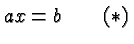where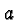and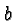are parameters and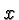is the variable whose value needs to be determined. There are three possible cases:

1.In this case dividing byon both sides ofgives the unique solution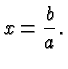The word unique in this context means there is a solution, and it's the only one.

2.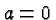and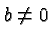. In this caseturns into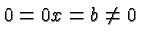. Since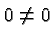is impossible there is no solution.

3.and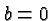. In that caseturns into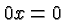which is true for all numbers. There are infinitely many solutions.

It is clear since we cover all possibilities above that it is impossible for theto have precisely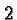, or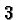, or any finite number, of solutions. Compare this for example with quadratic equations which may have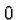,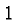, or, but never any other number of (real) solutions.

Next Consider two equations in two unknowns, let's say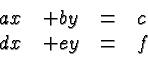Each of these two equations defines a line in the cartesian plane . All solutions are the coordinates of a point where the two lines intersect. There are again three possibilities.

1.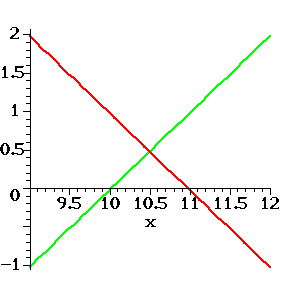Figure 1: Unique Solution of two equations in two unknowns.

The lines intersect in one point. There is a unique solution (i..e, the coordinates of that point). An example is provided by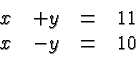The solution is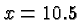and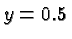, as shown in Figure 1.

2.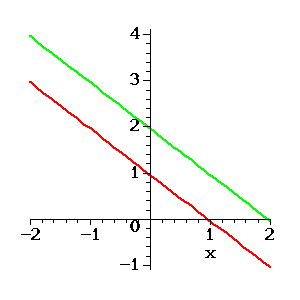Figure 2: No Solution of two equations in two unknowns.

The lines are parallel but distinct. They never intersect and there is no solution. An example is provided by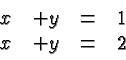Note that obviously the two equations contradict each other, nothing can simultaneously equaland.

3.Figure 3: Infinitely many solutions of two equations in two unknowns.

The lines are identical. Any point on the lines provides a solution. A trivial example can be obtained by writing the same equation twice. A less trivial example is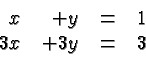The second equation follows from the first by multiplying on both sides with 3.

More than two Equations. Similar considerations apply to systems of more than two equations, but this is a subject beyond the scope of this class. You will learn more when you take a class on Linear Algebra.

### A Complicated Example

Suppose we want to find a quartic polynomial whose value equals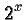for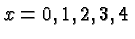. The purpose of this exercise might be to to approximateby a polynomial on a calculator that cannot evaluatefor non-integerdirectly. Approximating functions is a huge subject, here we just use this problem as an example for a more complicated linear system.

Let's write our quartic polynomial as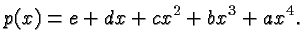We want it to satisfy the equations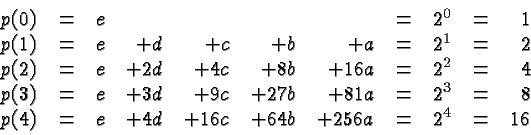This is a linear system of five equations in the five unknowns,,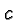,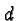, and.

The table below is set up as discussed except that whenever an entry isit is left blank to clarify the reduced systems.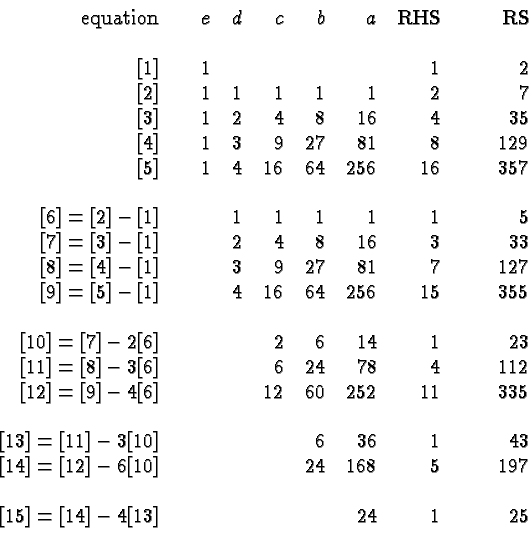Equation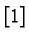is very special, it tells us right away that. We use that equation to eliminatefrom the remaining equations which gives us four equations (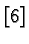through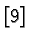) in the four unknowns,,and.

Equation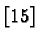is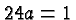which means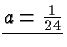. Substituting the value ofinto equation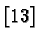gives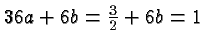which implies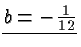. Substitutingandinto equationgives the equation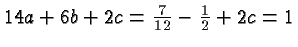which implies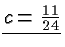. Finally, substituting,andinto equationgives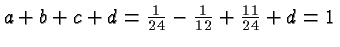which implies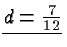.

Putting the underlined results together (and writing everything over the common denominator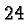) gives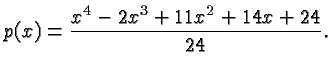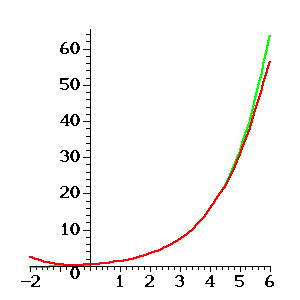Figure 4: A polynomial approximation of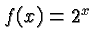.

Figure 4 shows the graph of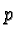(red) as well as the graph of(green). It is apparent thatis a good approximation of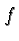in the interval fromto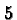. There are other and more effective ways of computing polynomials like. However, this example illustrates how Gaussian Elimination and Backward Substitution can be used to solve a linear system. In this particular linear system we were fortunate in that the elimination proceeded in a straightforward way without fractional arithmetic. It happens frequently that linear systems have a special structure that can be effectively exploited.

A final word on computing the row sums. They appear to be a waste of effort when the problem is all solved. However, when I first computed the entries in the above table I made several mistakes that I discovered immediately because of the row sums. There is a good chance you will save yourself a lot of time and aggravation by carrying them along in your own calculations.